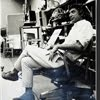## Eugene Ray SDSU 1970'sEugene Ray 1970's. photo credit: Tom Davis

## Tuesday, November 12, 2013

### NEW ORLEANS WORLD FAIR 2015/ radiant architecture prototypes

+WORLD'S FAIR 2015+
>how about building a new Worlds Fair<
>on the old Foucher-deBore plantation<
^^^^^^^^^^^^^^^^^^^^^^^^^^

+PERSPECTIVE 2013+
>researching and writing yesterday's blog was<
>joyful because it gave me a chance to visit<
(+FOUCHER-BORE'-GAYARRE+
+SAULET-GRUY-CAVAROC+
+RAY-STAIGG ETC ETC+)
>the milieu of our ancestors in what is now<
+TULANE UNIVERSITY+
>and Audubon Park where so much of our<
>life was spent in Radiant days of the early<
>pursuit of a Biotronic Architecture<
^^^^^^^^^^^^^^^^^^^^^^
+DNA ALCHEMY+
>synchronicity galvanizes vision<
>as i outline a radiant potential<
^^^^^^^^^^^^^^^^^^^
+DEDICATION+
>our mother was the daughter of a very<
+GEORGE STAIGG+
>talented cotton broker and grew up<
>in their New Orleans home at the<
+FOUCHER LAND+
>corner of Elenore and Perrier<
>streets near Tulane University<
^^^^^^^^^^^^^^^^^^

>as a theme for this fair would provide<
>opportunity to construct experimental<
+TENSEGRITY &+
+BIOTRONIC+
>structures from our research oeuvre<
^^^^^^^^^^^^^^^^^^^^^^^

+NEW CONSTRUCTION+
>methods would be demonstrated in front<
>of park patrons as the buildings fabric<
>would be woven as they watch<
^^^^^^^^^^^^^^^^^^^^^

+COSMIC CONSCIOUSNESS+
>would be emphasized at the fair w/ insight<
+VISIONARY DESIGN+
>building lighter, more economical<
>environmentally sound design<
^^^^^^^^^^^^^^^^^^^^

>would be shown of a residential nature<
>emphasizing economy, health and<
+BIOTRONICS+
>environmental strength factors<
^^^^^^^^^^^^^^^^^^^

+FIBER OPTIC+
>tensile structures would create a large<
+SYNERGETIC+
>expressive environ célébration<
^^^^^^^^^^^^^^^^^^^

+DISASTER RESISTANT+
>sculptural structure would be joined by<
>basic affordable VW like dome type
+HOUSING CONCEPTS+
>that can be constructed from kits<
^^^^^^^^^^^^^^^^^^

>of other dimensions (worm hole etc )<
+MULTI MEDIA+
>and back engineered technology<
>to be presented with care<
^^^^^^^^^^^^^^^^

+WORLDS FAIR 2015+
>into the<
^^^^^^^^^^^^
>it would be very rewarding to have<
>the researchers i have met at the<
+OPEN MINDS+
>conferences to aid programing<
^^^^^^^^^^^^^^^^^^^
+W/ MANY OF OUR TALENTED+
+SDSU-ED ALUM DESIGNERS+
^^^^^^^^^^^^^^^^^^^^^
eugene ray, mfa, architect
professor emeritus, sdsu